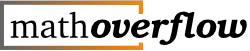0

(click on this box to dismiss)Q&A for professional mathematicians

``````-- http://blog.stackoverflow.com/2011/06/optimizing-for-pearls-not-sand/
declare @epoch datetime = '##epoch?2011-05-06##';

with sample_posts as (
select p.Id,
v.CreationDate posted,
1 Score,
case
when v.CreationDate < @epoch then
case
when PostTypeId = 1 then 'ten_question'
when PostTypeId = 2 then 'ten_answer'
end
when v.CreationDate > @epoch then
case
when PostTypeId = 1 then 'five_question'
when PostTypeId = 2 then 'five_answer'
end
end post_type
from PostsWithDeleted p
join Votes v on p.Id = v.PostId
where PostTypeId in (1,2)
and VoteTypeId = 3
)

select --count(*),
dateadd(week, datediff(week, @epoch, posted), @epoch) week,
sum(case when post_type = 'ten_question' then Score end)
- sum(case when post_type like 'ten_answer' then Score end) ten_vote,
sum(case when post_type = 'five_question' then Score end)
- sum(case when post_type like 'five_answer' then Score end) five_vote
from sample_posts
group by datediff(week, @epoch, posted)
having count(*) > 50
order by datediff(week, @epoch, posted)``````

### Enter Parameters

Options:
-Hold tight while we fetch your results
:records returned in :time ms:cached